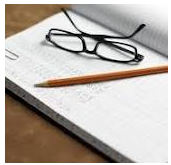Accounting ratios have deep relationship with balance sheet. There are lots of accounting ratios which are calculated with the help balance sheet's assets and liabilities. So, there is no any difficulty to calculate assets and liabilities on result values of accounting ratios. If we succeed to make balance sheet from accounting ratios, we can understand the structure of balance sheet and utilization of ratios for making it. Following are its main steps:

1st. To Calculate Missing Figure of Assets or Liabilities by Using  Accounting Ratios Formula

It is you logic, how will you reach to your required balance sheet item. For example. You need to calculate total creditor. This is current liability of balance sheet. First of all check which accounting ratio values are given. Analyze it.

a)  For example, you are given GP ratio and gross profit. On this basis, you will calculate sales and cost of goods sold. Indirectly, it will be helpful to you to reach the figure of purchases because cost of goods sold formula is dependent on opening stock, purchase and closing stock figures. Any one figure can easily be calculated.

b) Debtor collection period and creditor collection period are used for calculating the figure of sundry debtors and sundry creditors.

c) Capital turnover ratio and fixed assets turnover ratio will be helpful for calculating value of capital and fixed assets.

2nd. To Remember All the items of Balance Sheet

You should also remember all the items of balance sheet. Some items may easily be calculated by putting the value of all previous calculated items.

3rd. To Practice with Some Examples

You should study more and more practical examples which will be helpful to gain the knowledge of this. We are giving one example relating to this.

From the following detail, make out the balance sheet with as many details as possible:

1. Stock velocity = 6
2. Capital turnover ratio = 2
3. Fixed Assets Turnover = 4
4. Gross Profit Turnover = 20%
5. Debtor Velocity = 2 months
6. Creditors Velocity = 73 days
The gross profit was Rs. 60000. Reserve and surplus amounted to Rs. 20000.
Closing Stock was Rs. 5000
in excess of opening stock.

Solution:

1. Calculate sales

Gross profit ratio = Gross Profit / Sales X 100
Since G / P ratio is 20% and gross profit is Rs. 60000, sales are :
20 / 100 = 60000 / sales
20 sales = 60000 X 100
sales = 60000 X 100 / 20 = Rs. 3,00,000

2. Calculation of Purchases :

Purchases = Cost of goods sold + closing stock - opening stock
As G/ P ratio is 20%, so cost of goods is 100- 20 or say 80% .
Cost of goods sold = 300000 X 80 /100
= Rs. 240000
Purchase = 240000 + 5000 (As closing stock is Rs. 5000 more than the opening
stock)

3. Calculation of Stock:

Stock Turnover Ratio = Cost of goods sold / Average stock
6 = 240000 / average stock
6 average stock = 240000
average stock = 240000/ 6
average stock = 40000
average stock = opening stock + closing stock / 2
( Since closing stock is Rs. 5000 more )
or 40000 = 2 op. stock + 5000 / 2
or 80000 - 5000 = 2 op. stock
or opening stock = 75000 / 2 = Rs. 37500
Closing Stock = opening stock + 5000
= 37500 + 5000 = Rs. 42500

4. Calculation of Debtors :

Debtors Velocity = Total Debtors / Credit Sales X No. of months
or 2 = Total debtors / 300000 X 12 ( calculated in months)
Or
Total Debtors = 2 X 300000 / 12
Total Debtors = Rs. 50,000

5. Calculation of Creditors :

Creditors Velocity = Total Creditors / Credit Purchase X No. of Working days

73 = total creditors / 245000 X 365
73 X 245000 / 365 = total creditors
Total creditors = Rs. 49000

6. Calculation of Capital

Capital Turnover Ratio = Cost of Goods Sold / Capital
2 =  240000 / capital
capital = 240000 / 2 = 120000
Alternatively,
In case capital is taken to mean net worth, then net worth = Rs. 120000.
Or Share Capital + Reserve and Surplus = Rs. 120000
Share capital + 20000 = 120000
Share capital = 120000 - 20000 = 100000

7. Calculation of Fixed Assets

Fixed Assets Turnover Ratio = Cost of Goods Sold / Fixed Assets
Or 4 X Fixed Assets = 240000
Fixed Assets = 240000 / 4 = 60000

 Liabilities Amount Assets Amount Capital (6) Reserve and Surplus (given) Creditors (5) 100000 20000 49000 Fixed Assets ( 7) Closing Stock (3) Debtors (4) Cash ( Balancing figure) 60000 42500 50000 16500 169000 169000.

## \$type=three\$a=hide\$cm=hide\$s=hide\$show=/2019/06/10-steps-to-become-better-investor.html\$l=hide

Name

false
ltr
item
Accounting Education: How to Make Balance Sheet from Accounting Ratios
How to Make Balance Sheet from Accounting Ratios
http://2.bp.blogspot.com/-lvs4R5lsUrA/Tw2G0ByX6pI/AAAAAAAAHOI/qURPg1RiNgE/s200/bs.PNG
http://2.bp.blogspot.com/-lvs4R5lsUrA/Tw2G0ByX6pI/AAAAAAAAHOI/qURPg1RiNgE/s72-c/bs.PNG
Accounting Education
https://www.svtuition.org/2012/01/how-to-make-balance-sheet-from.html
https://www.svtuition.org/
http://www.svtuition.org/
http://www.svtuition.org/2012/01/how-to-make-balance-sheet-from.html
true
2410664366776677676
UTF-8